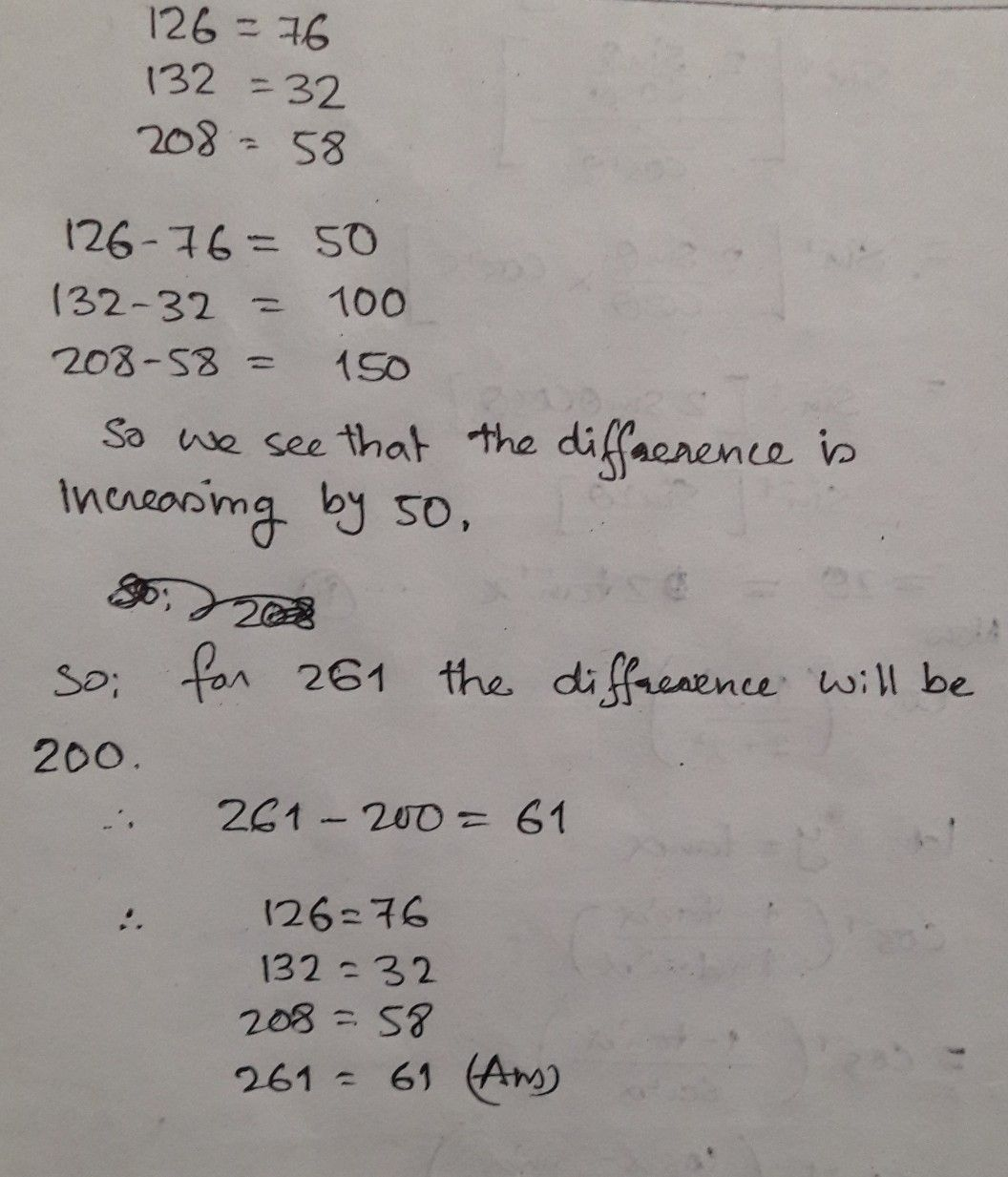Symbol
Problem$125=75$ $132=32$ $208=55$ $26=2$
10th-13th grade
Other
SolutionQanda teacher - ArghyajitStudent
yes, you are right. Keep it up??